Measures of Dispersion
Range, Standard Deviation, Variance, Mean Absolute Deviation

Problem:  For the data set {10, 12, 40, 35, 14, 24, 13, 21, 42, 30}, find the range, the standard deviation, the variance, and the mean absolute deviation to the nearest hundredth.

 A quick reminder before we begin the solution: In statistics, the population form is used when the data being analyzed includes the entire set of possible data.  The sample form is used when the data is a random sample taken from the entire set of data.  Check the "Standard Deviation" and "Variance" sections below for more information on how the calculator presents population versus sample forms.

To find the range:
To find the range:
Enter the data, as is, into L
1 You can enter the list on the home screen and "store" to L1, or you can go directly to L1 (2nd STAT, #1 Edit).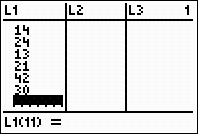Sort the list to quickly retrieve the highest and lowest values for the range. (2nd STAT, #2 SortA).  You can choose ascending or descending.

Read the high and low values from L1 for computing the range.
Range = 42 - 10 = 32.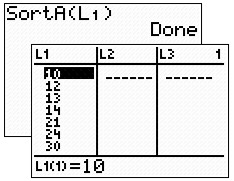OR:  To find the range:  Do not sort.  Simply type on the home screen using the min and max functions found under MATH → NUM #6 min and #7 max.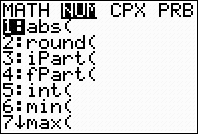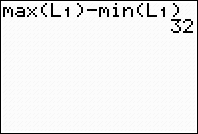Range = 32

To find standard deviation:

To find standard deviation:  Since this question deals with the complete set, we will be using "population" form, not sample form.

Go to one-variable stats for "population" standard deviation.    STAT CALC  #1 1-Var Stats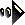NOTE! The standard deviations found in the CATALOG, stdDev, and also found by 2nd LIST → MATH #7 stdDev are both Sample standard deviations.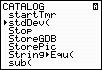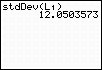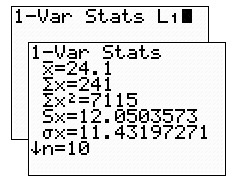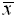= mean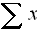= sum of the data= sum of squares of the data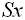= sample standard deviation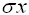= population standard deviation n = sample size (# of pieces of data)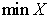= smallest data entry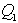= first quartile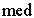= median (second quartile)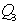= third quartile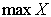= largest data entry

Population Standard Deviation = 11.43

FYI:  Using the lists, the calculator can simulate a spreadsheet style "by hand" computation of standard deviation.  Click here to see the spreadsheet-style approach.

To find variance:
To find variance:   The "population" variance is the square of the population standard deviation.

The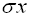symbol is under VARS - #5 Statistics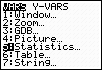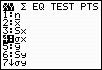NOTE!  The variance found in the CATALOG and also found by 2nd List → MATH #8 variance are both Sample variances.To find mean absolute deviation:
To find mean absolute deviation:
To calculate the mean absolute deviation you will have to enter the formula.NOTE!  Be sure that you have run 1-Var Stats (under STAT - CALC #1) first, so that the calculator will have computed.  Otherwise, you will get an error from this formula.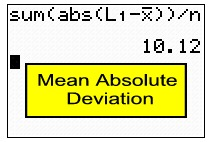and n are found under VARS #5 Statistics.  Sum and abs are quickly found in CATALOG.  Sum is also under 2nd LIST - MATH #5 sum.  abs is also under MATH - NUM #1abs.

OR:  To find mean absolute deviation:
A longer, but workable, solution can also be accomplished using the lists.  As stated above, run 1-Var Stats so the calculator will compute.  Now, go to L2 (STAT #1 EDIT) and move UP onto L2.  Type, at the bottom of the window, the portion of the formula that finds the difference between each data entry and the mean, using absolute value to make these distances positive.  Now, find the mean,, of L2 by using 1-Var Stats on L2, and read the answer of 10.12.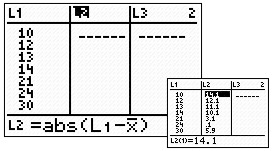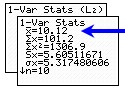Finding Your Way Around TABLE of  CONTENTS